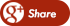﻿ Voltage vs. Current in a Resistor, Capacitor or Inductor:Back

## Voltage vs. Current in a Resistor, Capacitor or Inductor:

By Admin on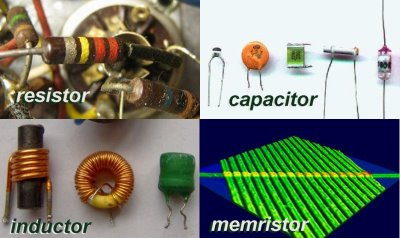# [Voltage vs. Current] in a Resistor, Capacitor or Inductor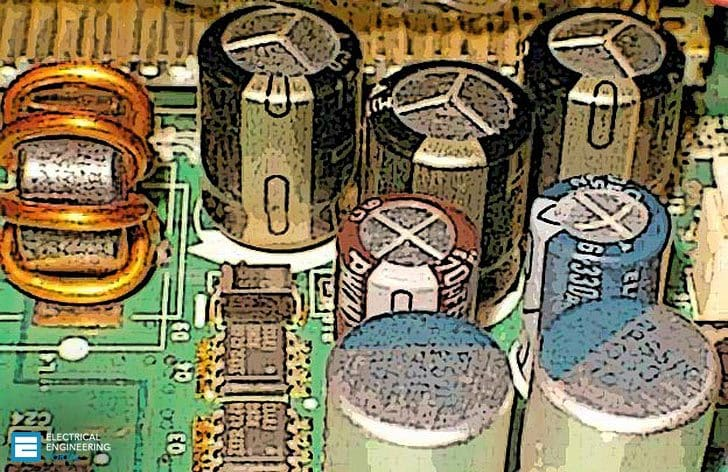Voltage vs. Current in a Resistor, Capacitor or Inductor

Elements in an electrical system behave differently if they are exposed to direct current as compared to alternating current. For ease of explanation, the devices have often been compared to similar every day items.

1. Resistors in electrical systems are similar to rocks in a stream of water.
2. A capacitor is comparable to a boat paddle inserted into the stream.
3. The action of inductor is similar to a coiled spring.

## DC Circuit Components

Let us first look at the simple case of a dc circuit composed of a constant EMF (battery) and the three basic elements and two configurations  (series/parallel).

## Resistors

As the current flows through the resistors, in the same way that water flows over rocks, it expends some of its energy. If the rocks in a stream were in the form of rapids, the stream would have considerable resistance. However, if the same amount of rocks were placed in a row across the stream, the overall resistance to current flow would be less.

The diagrams below illustrate the basic but underlying principle in the majority of electrical systems. The amount of potential required to force 1 Amp through 1 Ohm of resistance is 1 Volt (Ohms Law). This is often written as V=IR.

In the series circuit (on left), the same current flows through every resistor, but the applied voltage isdivided between them. In the parallel circuit (on right), the same voltage is applied to all resistors but the current divides between them.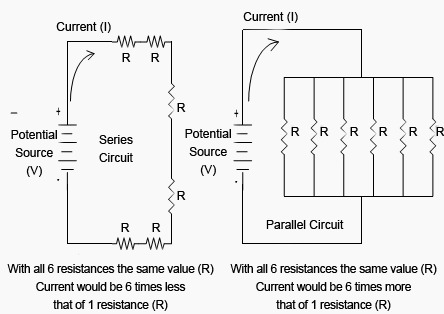Figure 1 – DC Circuit Resistance

For a series circuit the total resistance is stated as RTotal = R1 + R2 + (etc.).  In this exampleRTotal = 6R.

For a parallel circuit the total resistance is stated as 1/RTotal = 1/R1 + 1/R2 + (etc.). In this example 1/RTotal = 6/R or RTotal = R/6. Circuits containing a combination of series and parallel portions apply the same basic theory with more lengthy calculations.

## Capacitors

A capacitor, as previously described, is physicallymade of two conducting surfaces separated by an insulator. In an electrical circuit capacitors have both a steady state and transient effect on the circuit.

As the electrical conductors are not in physical contact, it will not, in the long-term pass direct current. The action is the same as placing a boat paddle against a stream of water – it blocks current flow. However when voltage is first applied to a capacitor current will flow until the capacitor is charged. This is a transient effect.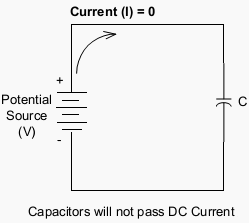Figure 2 – DC Circuit Capacitance Steady State Effect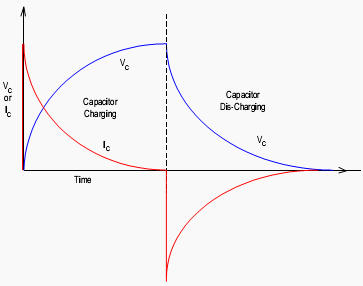Figure 3 – DC Circuit Capacitance Transient Effect

## Inductors

The inductor as illustrated in Figure 4 is similar to a coiled spring and in the steady, has no resisting capability. If a steady force is exerted on it, it can pass huge amounts of energy limited only by the supply capability or heaviness of the inductor. An inductor in a DC system has to be used with caution as it allowsunrestricted flow of energy and will drain the energy source or damage the inductor itself.

However, when voltage is first applied the inductor impedes current flow in the circuit.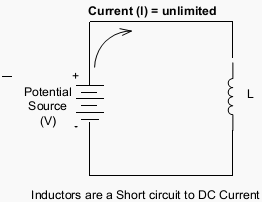Figure 4 – DC Circuit Inductance Steady State Effect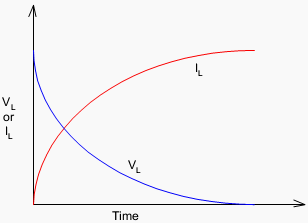Figure 5 – DC Circuit Inductor Transient Effect

## Transient Effects

The transient effects of capacitors and inductors are the result of stored energy in the electrical circuit. Energyis stored in two forms, as stored electrical charge in a capacitor and in the magneticfield in an inductor.

The amount of energy stored in an inductor depends on the current in the circuit; the amount of energy ina capacitor depends on the voltage across it. If the circuit conditions change so will the amount of energy stored in the component and a transient will occur.

One way of looking at the transient effects of these components is that inductors resist changes in current flow in a circuit and capacitors resist changes in voltage. In basic dccircuits inductors and capacitors are for the most part curiosities.

However, in an ac circuit the voltage and current are continuously changingso capacitor and inductors have a continuous effect on the circuit.

FOR MORE TOPICS:CLICK HERE